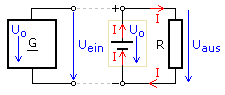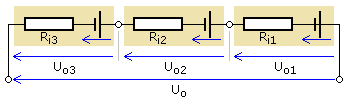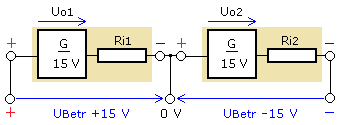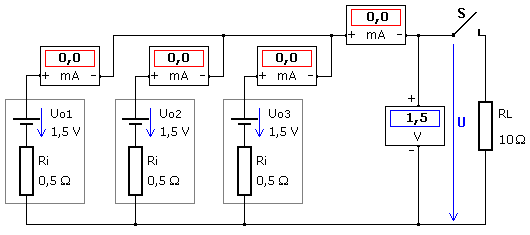# What is the source in a circuit

## The internal resistance of a voltage source

Charge carriers are present separately in a voltage source. This can be seen particularly clearly in the chemical systems of batteries and accumulators. In the case of generators, the separation takes place through the electromotive force, called EMF for short. The greater the separation force, the higher the tension generated. According to DIN 1323, the EMF is not one of the quantities that are used in the circuit. The voltage generated by the EMF is called the original voltage or source voltage. It can only be measured at the open connection terminals without loading the source.

The primary voltage generated by the EMF is considered to be ideal.
With currentless measurement, the terminal voltage is identical to the source or primary voltage.

If a load resistor is connected to a voltage source, a signal generator or a supply unit generally referred to as a source, electrical current flows. With increasing load, a larger current flows and the terminal voltage UKl decreases. The maximum current IK flows when the source is short-circuited, since the resistance value of the load then practically has the value 0 Ω. Electricity can only flow if the cause is electrical voltage. In any case, the laws of the electric circuit are valid. The rule of mesh applies to voltages in a power network. A part of the primary voltage U0 must therefore be converted within the voltage source. In the event of a short circuit, the current IL in the source generates a voltage Ui equal to the total source voltage. The cause of the externally measurable voltage loss can be explained by the internal resistance Ri of the generator.

The video clip can only be controlled with the help of the fade-in control bar. It shows that with increasing load, i.e. with lower values ​​of the load resistance, the terminal voltage decreases and at the same time the voltage at the internal resistance of the source increases. The source voltage is always the sum of the terminal voltage and the voltage drop across the internal resistance. The part highlighted in yellow is the equivalent circuit diagram of the voltage source. In practice, the voltage at the internal resistance cannot be measured directly. The internal resistance can be determined with a measuring diagram. With it all other values ​​can be calculated.

Every voltage generator or voltage source has an internal resistance.
The original voltage Uo is equal to the sum of the terminal voltage UKl and the voltage at the internal resistance Ui
The following applies to the terminal voltage: UKl = U0 - (IL Ri)

### The closed circuit

A closed circuit consists of a voltage source and a connected load, which is commonly referred to as a consumer. The voltage source can be a galvanic element or a battery. A battery is the interconnection of several galvanic elements or cells to form a larger unit. The voltage source can also be a generator or, in general, any signal source that provides electrical energy.

The source, the lines and the connected load form a closed circuit. Electric current flows, whereby the electrical energy stored in the source is converted into another form of energy in the consumer. The electrical energy is not consumed, but physically correctly converted into another form of energy, for example into thermal energy, light energy or mechanical energy.

The connection terminals of a voltage source have different electron occupation or potentials. They are measured at a reference potential that has the agreed value zero as the ground (earth) potential. The source voltage is calculated from the potential difference between the two connection poles. As long as it exists, electrical current can flow in a closed circuit.

The negative terminal has excess electrons and negative potential.
The positive terminal has an electron deficiency and positive potential.
The potential difference is the voltage between the terminals (terminal voltage).
The reference sense corresponds to the technical direction of current from plus to minus.A simple circuit is shown with a galvanic element, alternatively a DC voltage generator G, an ohmic resistor R and the lines. By definition, the cables have ideal, resistance-free properties. The arrows indicate the sense of reference for voltage and current. Input and output voltages are the same in this simple representation.

In the consumer arrow system, the voltage and current arrows on the consumer point in the same direction.
In the consumer arrow system, the voltage and current arrows in the voltage source point in opposite directions.

### The determination of the internal resistance according to the ΔU / ΔI method

In the voltage-current diagram of the video clip, the load characteristic shows the dependency of the terminal voltage on the drawn load current. This characteristic is also linear for load resistors with linear behavior. The internal resistance of the generator can be calculated directly from its gradient, see gradient triangle. This method can be used universally to determine the internal resistance. In the example, the internal resistance is calculated with ΔU = 3 V and ΔI = 0.3 A as Ri = 10 Ω

### The determination of the internal resistance using the method of half the terminal voltage

This measuring method may only be used without damage to a voltage source with a sufficiently high internal resistance. The load resistance is reduced until the terminal voltage has reached half the value of the source or open circuit voltage. The value of the load resistance is then the same as that of the internal resistance. In the video clip this is the case with a load resistance of 10 Ω. The terminal voltage, as well as the voltage at the internal resistance, is 5 V. Both resistors form a series circuit. The same current flows through them and they have the same resistance value with the same voltage drop.

### Voltage sources connected in series

An adjustable voltage source is not always available. Especially with battery-operated devices, the operating voltage is obtained by interconnecting several individual elements. When the elements are connected in series, their internal resistances also form a series connection. The total voltage is the sum of the individual voltages, paying attention to the polarity of the individual elements.When voltage sources are connected in series, the usable operating voltage increases.
The increase in internal resistance leads to a reduction in the short-circuit current.

A symmetrical voltage supply with positive and negative voltage is created by connecting two identical voltage sources in series with the reference point, the circuit ground between the two sources. The graphic shows a symmetrical voltage supply of ± 15 V generated with two identical sources and related to the circuit ground O V. The simple internal resistance of the source acts on the outside for each individual voltage.### The parallel connection of voltage sources

When connecting voltage sources in parallel, only voltage sources with the same original voltage and the same internal resistance should be connected together. The terminal voltage is then equal to the original voltage in no-load operation. Uterminal = U01 = U02 etc.
The total internal resistance is calculated according to Ohm's law of parallel connection of resistors. If n sources with the same internal resistance value are connected in parallel, the external internal resistance is: Ri tot = Ri / n

In the following switchable example, three 1.5 V elements of the same type are connected in parallel in the first two cases. When idling, the terminal voltage is 1.5 V as expected. There are no currents. The terminal voltage drops only slightly under load. Each element supplies the same proportion of the total electricity.In the last two cases, one of the elements is 'used up' or has been replaced by an element with a lower original voltage, a battery with 1.2 ... 1.4 V. A more strongly discharged (used) electrochemical cell less than 1.5 V also has a greater internal cell resistance. Its influence cannot be represented in the simulation. With elements with different open circuit voltages connected in parallel, the terminal voltage of the interconnection is slightly lower than with three identical cells with the same load. The partial currents show strong changes, with the branch current of the weak cell even becoming negative and flowing in the opposite direction. The last case shows the parallel connection of unequal cells for the unloaded idling. When the switch is open, no load current flows, but high equalizing currents still flow within the parallel circuit, which can lead to the rapid destruction of chemical primary cells and accumulators.

The parallel connection of voltage sources is used when high currents are required with a terminal voltage that is as constant as possible.
To prevent damaging equalizing currents, only voltage sources with the same original voltage and the same internal resistance may be connected in parallel.